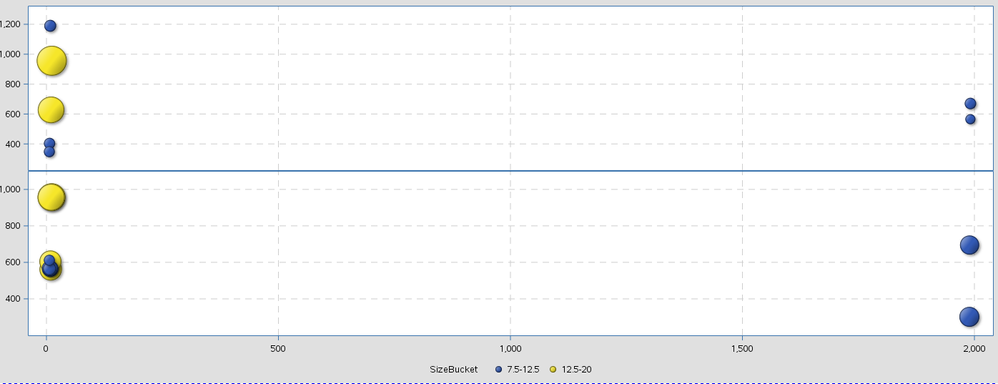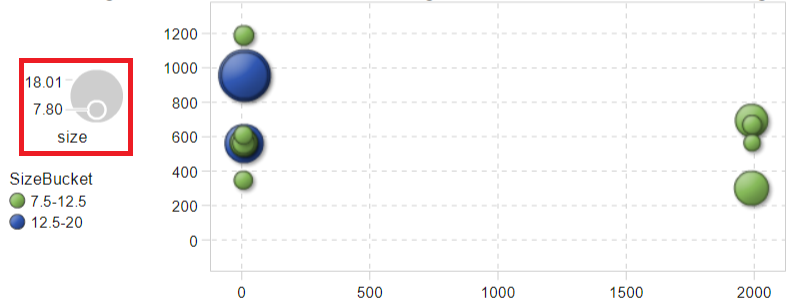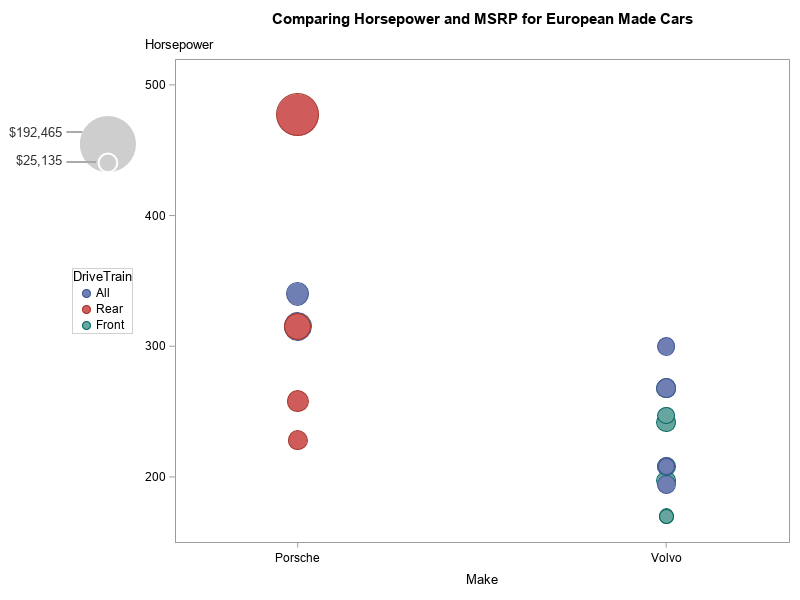## Bubble Plot Legend

Hello SAS Experts,

If someone can tell me how to have such a legend in the red square below (picture 2) in the bubble plot?

Size is a continuous variable, 7.80 and 18.01 are its minimum and maximum respectively.  I created a new category variable named SizeBucket based on size.

Right now what I have is picture 1 and I would like to have a result like picture 2.

Thanks,

Relax1 ACCEPTED SOLUTION

Accepted Solutions

## Re: Bubble Plot Legend

Currently, I believe the only way to get a size legend with sgplot bubble is to annotate it, and unfortunately that takes a bit of code.

Here's an example, you might be able to re-use ...

data my_data; set sashelp.cars (where=(origin='Europe' and make in ('Porsche' 'Volvo')));
run;

proc sql noprint;
select min(msrp) format=dollar10.0 into :minmsrp separated by ' ' from my_data;
select max(msrp) format=dollar10.0 into :maxmsrp separated by ' ' from my_data;
quit; run;

data anno_bubble_legend;
length function x1space y1space display fillcolor linecolor textcolor anchor \$50;

x1space='graphpercent'; y1space='graphpercent';

function='oval'; display='fill'; fillcolor='grayce';;
x1=13.5; y1=71.5; anchor='bottom';
height=45; width=45;
heightunit='pixel'; widthunit='pixel';
output;

function='oval'; display='outline'; linecolor='white';
x1=13.5; y1=71.5; anchor='bottom';
height=15; width=15;
heightunit='pixel'; widthunit='pixel';
output;

function='text'; anchor='right'; fillcolor=''; textcolor='gray33';
width=100; widthunit='percent';
x1=8.3; y1=78; label="&maxmsrp"; output;
x1=8.3; y1=73; label="&minmsrp"; output;

function='line'; linecolor='grayaa';
x1space='graphpercent'; y1space='graphpercent';
x2space='graphpercent'; y2space='graphpercent';
x1=8.3; y1=78; x2=x1+2; y2=y1; output;
x1=8.3; y1=73; x2=x1+3.7; y2=y1; output;

run;

title 'Comparing Horsepower and MSRP for European Made Cars';

ods graphics / width=800px height=600px noborder;

bubble x=make y=horsepower size=msrp / group=drivetrain;
xaxis offsetmin=.2 offsetmax=.2;
yaxis labelpos=top;
keylegend / position=left;
run;4 REPLIES 4

## Re: Bubble Plot Legend

Is this using SAS VA? If so, I can move this post to that forum specifically.

## Re: Bubble Plot Legend

The picture 2 was created using SAS VA, but I was trying to create a same plot in the SAS EG (picture 1):smileyhappy:

## Re: Bubble Plot Legend

Currently, I believe the only way to get a size legend with sgplot bubble is to annotate it, and unfortunately that takes a bit of code.

Here's an example, you might be able to re-use ...

data my_data; set sashelp.cars (where=(origin='Europe' and make in ('Porsche' 'Volvo')));
run;

proc sql noprint;
select min(msrp) format=dollar10.0 into :minmsrp separated by ' ' from my_data;
select max(msrp) format=dollar10.0 into :maxmsrp separated by ' ' from my_data;
quit; run;

data anno_bubble_legend;
length function x1space y1space display fillcolor linecolor textcolor anchor \$50;

x1space='graphpercent'; y1space='graphpercent';

function='oval'; display='fill'; fillcolor='grayce';;
x1=13.5; y1=71.5; anchor='bottom';
height=45; width=45;
heightunit='pixel'; widthunit='pixel';
output;

function='oval'; display='outline'; linecolor='white';
x1=13.5; y1=71.5; anchor='bottom';
height=15; width=15;
heightunit='pixel'; widthunit='pixel';
output;

function='text'; anchor='right'; fillcolor=''; textcolor='gray33';
width=100; widthunit='percent';
x1=8.3; y1=78; label="&maxmsrp"; output;
x1=8.3; y1=73; label="&minmsrp"; output;

function='line'; linecolor='grayaa';
x1space='graphpercent'; y1space='graphpercent';
x2space='graphpercent'; y2space='graphpercent';
x1=8.3; y1=78; x2=x1+2; y2=y1; output;
x1=8.3; y1=73; x2=x1+3.7; y2=y1; output;

run;

title 'Comparing Horsepower and MSRP for European Made Cars';

ods graphics / width=800px height=600px noborder;

bubble x=make y=horsepower size=msrp / group=drivetrain;
xaxis offsetmin=.2 offsetmax=.2;
yaxis labelpos=top;
keylegend / position=left;
run;## Re: Bubble Plot Legend

Awesome!  Thanks a lot!

Discussion stats
• 4 replies
• 1185 views
• 3 likes
• 3 in conversation